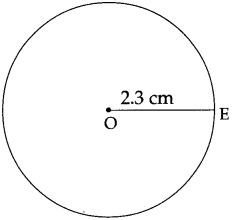Balbharti Maharashtra Board Class 5 Maths Solutions Chapter 7 Circles Problem Set 28 Textbook Exercise Important Questions and Answers.

## Maharashtra State Board Class 5 Maths Solutions Chapter 7 Circles Problem Set 28

Question 1.
Draw circles with the radii given below.

(1) 2 cm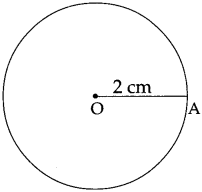(2) 4 cm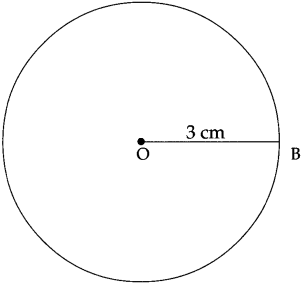(3) 3 cm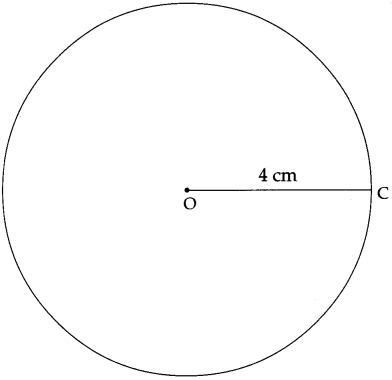Question 2.
Draw a circle of any radius. Show one diameter, one radius and one chord on that circle.
In a circle, P is the centre.
AB is a diameter.
MN is a chord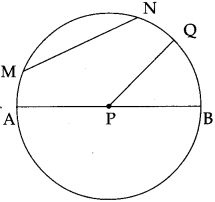Study the circle given alongside. Think over the following questions.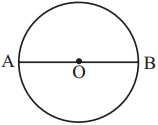• Which are the radii in the circle?
• How many radii make up diameter AB?
• If the length of one radius is 3 cm, what is the length of the diameter?
• How long is the diameter as compared to the radius?The diameter of a circle is twice the length of its radius.

• If another diameter CD is drawn on the same circle, will its length be the same as that of AB?

All the diameters of a circle are of the same length.

Test 1 :
Measure the diameters and radii of the circles given below with a ruler and verify the relationship between their lengths.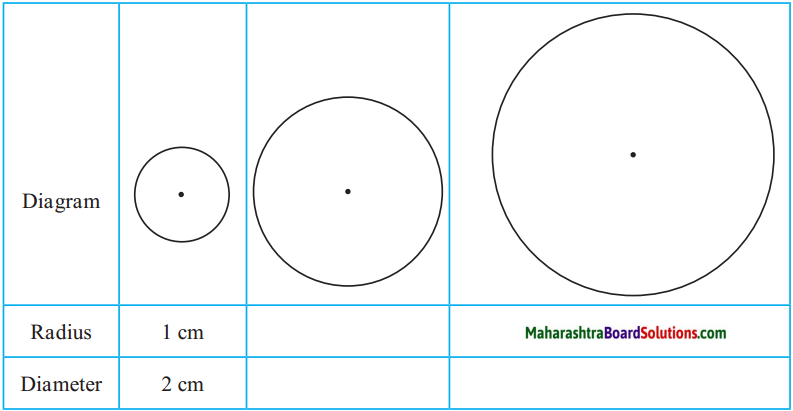Test 2 :
1. Draw a circle on a piece of paper and cut it out.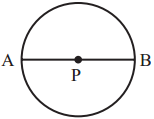2. Name the centre of the circle P.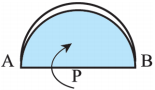3. Draw the diameter of the circle and name it AB. Note that PA and PB are radii of the circle.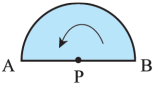4. Fold the circular paper along AB as shown in the picture.Fold the paper at P in such a way that point B will fall on point A. Radius PB falls exactly on radius PA. In other words, they coincide.

From this, we can see that every radius of a circle is half the length of its diameter.

Question 1.
Draw circles with the radii given below:

(1) 1.5 cm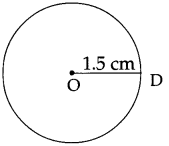(2) 2.3 cm